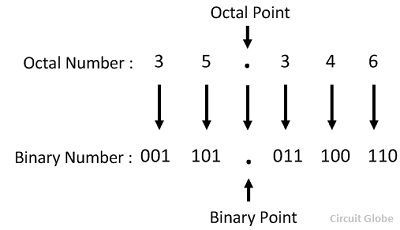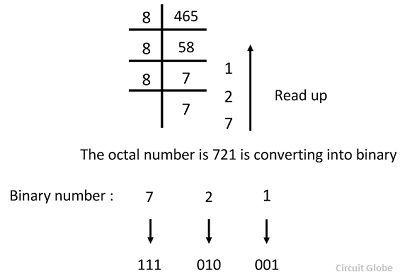# Octal to Binary & Binary to Octal Conversion Methods

The base of the octal number system is 8 because it consists the digit from 0 – 7. The octal number system is converting into binary number system by grouping the binary digit in the group of three from the right of the binary number. On the other hand, the base of the binary digit is 2 and it consists only two digits 0 and 1. The octal number can easily be converted into binary by the help of the method explained below.

## Octal to Binary Conversion

The base of the octal number system (8) is the third power of base of a binary system (2), the interconversion of octal and a binary number is very simple and direct as explained below.

Let converting the octal number 35.346 to its equivalent binary numberThus the required binary number is 011 101. 011 100 110.

### Binary to Octal Conversion

The conversion of binary to octal is a reversal of the above procedure. For example, the binary number 010100111.100011 can be converted into an octal number by first written the bits in the group of three and then awarding the decimal number of to each of the group of three bits as shown below.Thus the required octal number is 257.43

### Second Method of Binary to Octal Conversion

In this method, the large decimal number is first converted into an octal number and then to a binary number instead of direct conversion of decimal to binary number as shown in the following examples.

Suppose it is required to convert the decimal number 465 into a binary number. Let us find an octal number of 465 using the octal dabble method.Thus the binary equivalent number is 111 010 001.